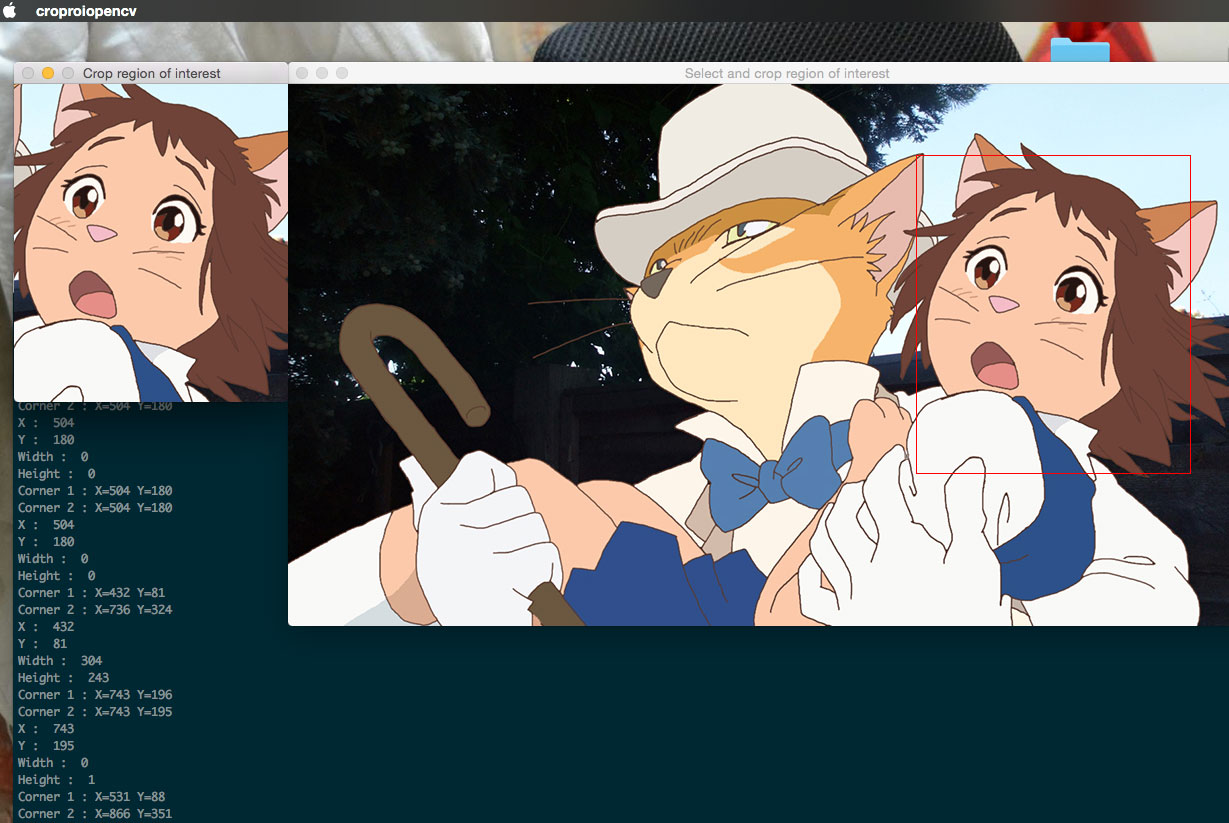# Golang : Select region of interest with mouse click and crop from image

Tags :

Just a short Golang OpenCV program that allows the user to select a region of interest on an image with mouse click. The selected rectangular region of interest will be cropped and displayed on another window.

An example screen shot:Here you go!

`````` package main

import (
"fmt"
"github.com/lazywei/go-opencv/opencv"
"math"
"os"
)

var (
win = new(opencv.Window)
cropWindow = new(opencv.Window)
corner1 = new(opencv.Point)
corner2 = new(opencv.Point)
pt = new(opencv.Point)
leftDown = false
leftUp = false
image = new(opencv.IplImage)
redColor = opencv.NewScalar(0, 0, 255, 255) // (blue, green, red, alpha)
)

func mouseCallBack(event, x, y, flags int, param ...interface{}) {

// if mouse left button is pressed, record the positions and save to corner1
if event == opencv.CV_EVENT_LBUTTONDOWN {
leftDown = true
corner1.X = x
corner1.Y = y
fmt.Printf("Corner 1 : X=%d Y=%d\n", corner1.X, corner1.Y)
}

if event == opencv.CV_EVENT_LBUTTONUP {
leftUp = true
corner2.X = x
corner2.Y = y
fmt.Printf("Corner 2 : X=%d Y=%d\n", corner2.X, corner2.Y)
}

// draw a red rectangle box when left mouse button is pressed and drag
if (leftDown == true) && (leftUp == false) {
pt.X = x
pt.Y = y
roiImage := image.Clone()
opencv.Rectangle(roiImage, *corner1, *pt, redColor, 1, 4, 0)
win.ShowImage(roiImage)
}

// crop image when both corners are populated
if (leftDown == true) && (leftUp == true) {

cropBoxWidth := math.Abs(float64(corner1.X) - float64(corner2.X))
cropBoxHeight := math.Abs(float64(corner1.Y) - float64(corner2.Y))
cropBoxX := math.Min(float64(corner1.X), float64(corner2.X))
cropBoxY := math.Min(float64(corner1.Y), float64(corner2.Y))

fmt.Println("X : ", cropBoxX)
fmt.Println("Y : ", cropBoxY)
fmt.Println("Width : ", cropBoxWidth)
fmt.Println("Height : ", cropBoxHeight)

// sanity check on input parameters
// ignore if user just click on the image
// i.e width and height equal zero

if (cropBoxWidth != 0) && (cropBoxHeight != 0) {
cropImage := opencv.Crop(image, int(cropBoxX), int(cropBoxY), int(cropBoxWidth), int(cropBoxHeight))
cropWindow.Resize(int(cropBoxWidth), int(cropBoxHeight))
cropWindow.ShowImage(cropImage)
cropImage.Release()
}
leftDown = false
leftUp = false
}

}

func main() {

if len(os.Args) != 2 {
fmt.Printf("Usage : %s <image filename>\n", os.Args)
os.Exit(0)
}

imageFileName := os.Args

fmt.Println("Press ESC key to quit")

if image == nil {
}
defer image.Release()

win = opencv.NewWindow("Select and crop region of interest")
win.Move(100, 100) // so that this window does not hide the crop window
defer win.Destroy()

cropWindow = opencv.NewWindow("Crop region of interest")
defer cropWindow.Destroy()

win.SetMouseCallback(mouseCallBack)
win.ShowImage(image)

for {
key := opencv.WaitKey(20)
if key == 27 {
os.Exit(0)
}
}

os.Exit(0)
}
``````

References:

https://socketloop.com/tutorials/golang-put-utf8-text-on-opencv-video-capture-image-frame

https://github.com/lazywei/go-opencv/blob/master/samples/crop.go

Tags :